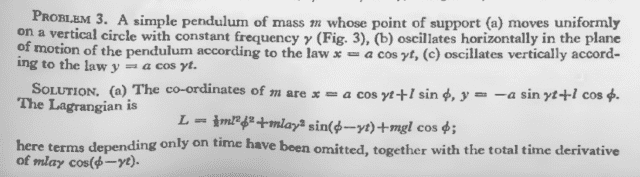# Lagrangian of a driven pendulum (Landau problem)

Robin04

## Homework Statement

There's the following problem (the task is to construct the Lagrangian) in the first Landau (part a):My problem is that I don't understand what did we omit exactly and why.

## The Attempt at a Solution

I did the calculation myself (even checked with Mathematica) and got the following result:
##L= \frac{1}{2}ml^2\dot{\phi^2} + mla\gamma sin(\phi - \gamma t)\dot{\phi} +mgl cos(\phi) + \frac{1}{2}ma^2\gamma^2##
The solution says that we omit the terms that depend explicitly only on time, but there's no such term here. They omitted the last term for sure, but that's a constant, it has no time-dependence.

Also, the time derivative of that cosine term:
##\frac{d}{dt}[mla\gamma cos(\phi - \gamma t)]=...=-mla\gamma sin(\phi - \gamma t)\dot{\phi}+mla\gamma^2 sin(\phi-\gamma t)##
Landau seems to only leave the first term here, but why? The 'total derivative' (as he mentions) should mean both terms together, what makes the first one negligable?

#### Attachments

Homework Helper
Gold Member
##L= \frac{1}{2}ml^2\dot{\phi^2} + mla\gamma sin(\phi - \gamma t)\dot{\phi} +mgl cos(\phi) + \frac{1}{2}ma^2\gamma^2##
The solution says that we omit the terms that depend explicitly only on time, but there's no such term here. They omitted the last term for sure, but that's a constant, it has no time-dependence.
There's a term associated with the gravitational potential energy that depends only on time.

Also, the time derivative of that cosine term:
##\frac{d}{dt}[mla\gamma cos(\phi - \gamma t)]=...=-mla\gamma sin(\phi - \gamma t)\dot{\phi}+mla\gamma^2 sin(\phi-\gamma t)##
Landau seems to only leave the first term here, but why? The 'total derivative' (as he mentions) should mean both terms together, what makes the first one negligable?

Note that the middle term of the right-hand side of your expression for ##L## is not quite the same as Landau's middle term for ##L##. You can use your expression for ##\frac{d}{dt}[mla\gamma cos(\phi - \gamma t)]## to express your middle term of ##L## in terms of Landau's middle term.

•Robin04
Robin04
There's a term associated with the gravitational potential energy that depends only on time.
I realized that I didn't really think about the potential energy just accepted it as it is.
So let's call ##d## the vertical displacement of the point of support relative to its lowest possible position, and let's put the zero-potential at the lowest possible position of the pendulum. Then the height of the mass above this level is ##h=d+l(1-cos(\phi))##, and ##d=a(1-sin(\gamma t))##
If I leave the sine term as it only depends explicitly on time I still have ##U=mga+mgl(1-cos\phi)##
I assume I have to leave all the constants too, and this seems so trivial that Landau doesn't even mention it, but I don't really understand why can we do this. I suppose this was also the case with the ##\frac{1}{2}ma^2\gamma^2## term.
Note that the middle term of the right-hand side of your expression for LLL is not quite the same as Landau's middle term for LLL. You can use your expression for ddt[mlaγcos(ϕ−γt)]ddt[mlaγcos(ϕ−γt)]\frac{d}{dt}[mla\gamma cos(\phi - \gamma t)] to express your middle term of LLL in terms of Landau's middle term.
Oh, so that's what he means by leaving the total derivatives. But I don't understand this either. Why can we leave the total derivatives? I had classical mechanics this semester and my teacher neglected lots of things too, but I don't really see why are the neglected terms less important than the others. What's so special about only time dependent, total derivative or constant terms?
I haven't noticed it, thank you! However, they don't discuss why are those terms neglected.

Homework Helper
Gold Member
What's so special about only time dependent, total derivative or constant terms?
Such terms do not affect the equations of motion (i.e., the Euler-Lagrange equations given by equation (2.6) in Landau on page 3). Any two Lagrangians that differ only by such terms, will produce the same equations of motion. So, you can neglect such terms in the Lagrangian when deriving the equations of motion.

It should be fairly clear by inspection of the Euler-Lagrange equations that terms in the Lagrangian that are either constant or depend only on time will not affect the equations of motion.

As far as terms that are total derivatives, see Landau's explanation on page 4 in the last paragraph before section 3.

•Delta2 and Robin04
Robin04
Such terms do not affect the equations of motion (i.e., the Euler-Lagrange equations given by equation (2.6) in Landau on page 3). Any two Lagrangians that differ only by such terms, will produce the same equations of motion. So, you can neglect such terms in the Lagrangian when deriving the equations of motion.

It should be fairly clear by inspection of the Euler-Lagrange equations that terms in the Lagrangian that are either constant or depend only on time will not affect the equations of motion.

As far as terms that are total derivatives, see Landau's explanation on page 4 in the last paragraph before section 3.
It's all clear now, thank you very much!